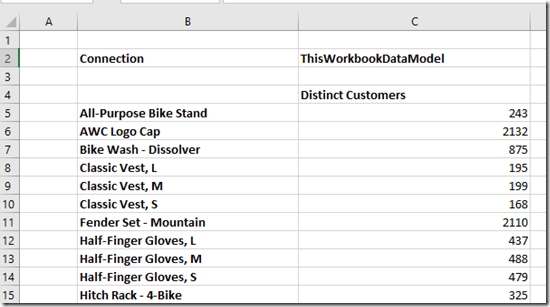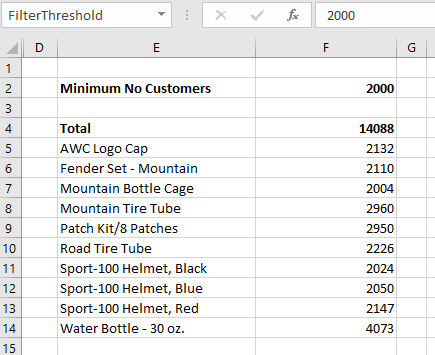# Excel Cube Functions, Dynamic Arrays And Lambdas, Part 2: Returning Tables

In the first post in this series I showed how to use the new Excel Lambda helper functions to return an array containing all the items in a set. That isn’t very useful on its own, so in this post I’ll show you how to generate an entire dynamic table using Excel cube functions and Lambda helper functions.

In this post I’ll be using the same source data as in my previous post: a table containing sales data with just two columns.

With this table added to the Excel Data Model/Power Pivot, I created two measures:

I then created created two sets using CubeSet containing the sets of Products (in cell B2 of my worksheet) and Measures (in cell B4) to use in my table:

```=CUBESET("ThisWorkbookDataModel", "[Sales].[Product].[Product].MEMBERS", "Product Set")

=CUBESET("ThisWorkbookDataModel", "{[Measures].[Sales Amount], [Measures].[Forecast Sales]}", "Measure Set")
```

Here are the formulas shown in the worksheet:

And here’s the output – remember you only see the text in the third parameter displayed in the cell:

Now, here’s the fun part – a single formula that takes these sets and builds a table with the Measures on columns and the Products on rows:

```=MAKEARRAY(
CUBESETCOUNT(B2)+1,
CUBESETCOUNT(B4)+1,
LAMBDA(r,c,
SWITCH(
TRUE(),
AND(r=1,c=1),
"",
c=1,
CUBERANKEDMEMBER("ThisWorkbookDataModel",\$B\$2,r-1),
r=1,
CUBERANKEDMEMBER("ThisWorkbookDataModel",\$B\$4,c-1),
CUBEVALUE("ThisWorkbookDataModel",
CUBERANKEDMEMBER("ThisWorkbookDataModel",\$B\$2,r1),
CUBERANKEDMEMBER("ThisWorkbookDataModel",\$B\$4,c-1)
)
)
)
)
```

Here’s what this formula returns:

How does this work? Going through the MakeArray function step-by-step:

• The first two parameters specify that the output will be an array with one more row than there are items in the Product set and one more column than there are items in the Measures set.
• The third parameter returns a Lambda that is called for every cell in this array. This Lambda contains a Switch with the following conditions:
• For the top-left cell in the array, return a blank value
• In the first column, use the CubeRankedMember function to return the Products on the rows of the table
• In the first row, use the CubeRankedMember function to return the Measures on the columns of the table
• In the body of the table, use the CubeValue function to return the values

Here’s a slightly more ambitious version that returns the same table but adds a total row to the bottom:

```=
LET(
NumberOfRows,
CUBESETCOUNT(B2)+2,
NumberOfColumns,
CUBESETCOUNT(B4)+1,
MAKEARRAY(
NumberOfRows,
NumberOfColumns,
LAMBDA(r,c,
SWITCH(
TRUE(),
AND(r=1,c=1),
"",
AND(r=NumberOfRows,c=1),
"Total",
r=NumberOfRows,
CUBEVALUE("ThisWorkbookDataModel",
\$B\$2,
CUBERANKEDMEMBER("ThisWorkbookDataModel",\$B\$4,c-1)),
c=1,
CUBERANKEDMEMBER("ThisWorkbookDataModel",\$B\$2,r-1),
r=1,
CUBERANKEDMEMBER("ThisWorkbookDataModel",\$B\$4,c-1),
CUBEVALUE("ThisWorkbookDataModel",
CUBERANKEDMEMBER("ThisWorkbookDataModel",\$B\$2,r-1),
CUBERANKEDMEMBER("ThisWorkbookDataModel",\$B\$4,c-1))
)
)
)
)
```

Two extra things to note here:

• This is a great example of a complex formula where the new Excel Let function can be used to improve readability and prevent the same value being evaluated twice.
• The values in the Total row are calculated in the Excel Data Model, not on the worksheet, by using the CubeSet function inside the CubeValue function. This means that the totals will be consistent with what you see in a PivotTable and therefore correct

This is still very much a proof-of-concept. I need to look at the performance of this approach (it may not be optimal and may need tuning), and I’m not sure how a table like this could be formatted dynamically (especially the Total row). It is exciting though!

# Excel Cube Functions, Dynamic Arrays And Lambdas, Part 1: Getting All The Items From A Set

After my recent post on using Office Scripts and cube functions to generate Excel reports from Power BI data, Meagan Longoria asked me this question on Twitter:

To which I can only reply: guilty as charged. I have always loved the Excel cube functions even though they are maybe the least appreciated, least known and least used feature in the whole Microsoft BI stack. They have their issues (including sometimes performance) but they are great for building certain types of report in Excel that can’t be built any other way.

Anyway, the recent addition of new Lambda helper functions to Excel has made me particularly happy because they can be used with cube functions to overcome some limitations that have existed since cube functions were first released in Excel 2007, and to do some other cool things too. In this series of posts I’m going to explore some of the things they make possible.

Let’s start with something simple. In Excel, the CubeSet function can be used to return an (MDX) set of items. This set is stored in a single cell, though, and to extract each item into a cell on your worksheet you need to use the CubeRankedMember function. For example, let’s say I have a table called Sales on my worksheet:

…that is then loaded into the Excel Data Model (aka Power Pivot – although this works exactly the same if I use a Power BI dataset, Azure Analysis Services or SQL Server Analysis Services as my source):

What you can then do is use the CubeSet function to create a set of all the products like so:

```=CUBESET("ThisWorkbookDataModel", "[Sales].[Product].[Product].MEMBERS", "Product Set")
```

…and then use the CubeRankedMember function to put each individual item of the set into a cell. Here’s a simple example worksheet, first with the formulas showing and then the results:

This example shows the fundamental problem that has always existed with CubeRankedMember though: in order to show all the items in a set you need to know how many items there are in advance, and populate as many cells with CubeRankedMember formulas as there are items. In this case see how the range B4:B6 contains the numbers 1, 2 and 3; these numbers are used in the formulas in the range C4:C6 to get the first, second and third items in the set.

If a fourth product was added to the table, however, it would not appear automatically – you would have to add another cell with another CubeRankedMember formula in it manually. I’ve seen some workarounds but they’re a bit hacky and require you to know what the maximum possible number of items in a set could ever be. Indeed that’s always been one of the key differences between cube functions and PivotTables: cube functions are static whereas PivotTables can grow and shrink dynamically when the data changes.

The new MakeArray function in Excel provides a really elegant solution to this problem: you can now write a single formula that returns a dynamic array with all the items in the set in. Assuming that the same CubeSet exists in B2 as shown above, you can do the following:

```=MAKEARRAY(CUBESETCOUNT(\$B\$2), 1, LAMBDA(r,c,CUBERANKEDMEMBER("ThisWorkbookDataModel",Sheet3!\$B\$2,r)))
```

Here’s the output:

Notice how the formulas in cell B4 returns an array that contains all three items in the set into the range B4:B6.

How does this work?

1. The CubeSetCount function is used to get the number of items in the CubeSet in B2.
2. The MakeArray function is then used to create an array with the number of rows returned by CubeSetCount and one column
3. In the third parameter of MakeArray the Lambda function is used to return a function that wraps CubeRankedMember, which is then called with the current row number of the array

The nice thing about this is that when more products are added to the Sales table they automatically appear in the output of the MakeArray formula in B4. So, for example, with two more products added to the Sales table like so:

Here’s the new output of the formula, showing the two new products returned in the array automatically:

This is not very useful on its own though. In my next post I’ll show you how this can be used to build a simple report.

# Excel Dynamic Arrays And The CubeSet Function

Among all the exciting announcements made at Ignite last week, one you may have missed (even in all the Excel-related announcements here) was that dynamic arrays have finally reached GA. Ever since they were announced I’ve been interested in how they can be used with Excel cube functions, which allow you to get data from Analysis Services, Power BI and the Excel Data Model/Power Pivot into cells on the worksheet, and I’ve blogged about this once already. Even though right now the CubeValue function can’t be used with dynamic arrays – alas – there are still some interesting ways dynamic arrays and cube functions can be used together and in this post I’ll show you another one I’ve found.

Say you have the following set of tables in the Excel Data Model:And the following measure that counts the number of customers who bought something:

```Distinct Customers:=DISTINCTCOUNT('Internet Sales'[CustomerKey])

```

Let’s also say you have on a worksheet the following table of data showing the number of distinct customers broken down by product, created using the CubeMember and CubeValue functions:Here are the formulas for this table:So far, all very straightforward. Now let’s say you want to use a dynamic array to filter the rows in this table so you only see the products that have more than a certain number of distinct customers, and you want to see a grand total for this filtered list of customers, like so:Here are the formulas to achieve this:To explain what’s going on here:

• Cell C2 contains the text “ThisWorkbookDataModel”, the name of the connection to the Excel Data Model. Doing this makes the other Excel formulas here much easier to read!
• Cell F2 – which I’ve turned into a named range called FilterThreshold – contains the number of customers which a product must exceed to be shown.
• Cell E5 contains the dynamic array Filter function that filters the rows shown in the first two screenshots above and only returns the products that exceed the threshold. The formula is:
FILTER(B5:B134,C5:C134>FilterThreshold)
• Cell E6 does something similar to E5 but returns the number of distinct customers for each product. If all I wanted was the products and the number of distinct customers I wouldn’t have needed two separate formulas, I could have used one, but I need to add the grand total too…
• Cell E4 contains the formula:
CUBESET(\$C\$2, E5#, “Total”)
This creates a set from the spill reference E5#, which contains the cells containing CubeMember formulas returned by the Filter function. For some reason CubeSet returns an error if you try to use a dynamic array formula in its second parameter but using a spill reference works ok; this is why I had to use separate formulas in E5 and E6.
• Finally, cell F4 contains the formula that returns the total distinct count for all products returned in the filtered list using CubeValue and the set created in cell E4:
CUBEVALUE(\$C\$2, “[Measures].[Distinct Customers]”, E4)
Since this is a distinct count the only way to get the correct value here is using CubeSet, CubeValue and the DAX measure – there would be no way to calculate the correct value using Excel formulas. The same could be said of almost any other DAX measure that wasn’t a simple aggregation.